# How to Graph a Parabola in Vertex Form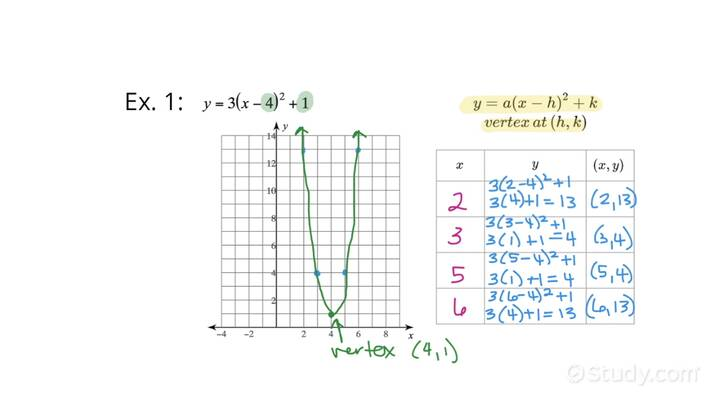An error occurred trying to load this video.

Try refreshing the page, or contact customer support.

• 00:04 How to graph a…
• 03:40 How to graph a…
Dana Hansen, Cassidy Holmes
• Instructors
Dana Hansen

Dana has taught high school mathematics for over 9 years. She has a masters in mathematics education from CU Denver. She is certified to teach both mathematics and physics in both Colorado as well as Iowa. She loves getting outdoors as much as possible hopefully with her two dogs. Dana has tutored with study.com for over a year, and loves the opportunity to work one on one with students to help them develop their content knowledge.

•
Cassidy Holmes

Cassidy Holmes has taught math for grades 7th-12th and has tutored many of students in math as well. She has a bachelors degree in Mathematics and a minor in Secondary Education from Louisiana Tech University. She also has successfully passed required Praxis exams for her teaching certification.

## How to Graph a Parabola in Vertex Form

Step 1: Use the vertex form provided to determine the vertex of the function. Graph this point. The general vertex form of a function is {eq}f(x)=a(x-h)^2+k {/eq}. The values that are in the place of {eq}h {/eq} and {eq}k {/eq} give us the vertex of the function. The vertex will be at the point {eq}(h,k) {/eq}.

Step 2: Create a table with two {eq}x {/eq} values that are less than the {eq}x {/eq} value of the vertex and two {eq}x {/eq} values that are greater than the {eq}x {/eq} value of the vertex. This would give a total of five values of {eq}x {/eq} including the vertex.

Step 3: Solve for the corresponding values of {eq}f(x) {/eq} using the chosen values of {eq}x {/eq} to finish the table.

Step 4: Graph the points from the table and connect these points with a smooth curve.

## How to Graph a Parabola in Vertex Form Vocabulary

Vertex Form of a Quadratic Function: The equation {eq}f(x)=a(x-h)^2+k {/eq} is the vertex form of a quadratic function. When a quadratic function is given in this form we can immediately see the coordinates of the vertex of the function. The vertex will be given by {eq}(h,k) {/eq}.

Vertex: This is either the highest point or the lowest point of a parabola. If the parabola opens up the vertex will be the lowest point, if the parabola opens down the vertex will be the highest point. The vertex is the center of the parabola, the left of the vertex will be a mirror image of the right of the vertex.

So, let's try using these steps to graph a parabola in vertex form, in the following two examples.

## How to Graph a Parabola in Vertex Form: Example 1

Graph the function {eq}f(x)=-3(x+2)^2+4 {/eq}

Step 1: Use the vertex form provided to determine the vertex of the function. Graph this point.

The general vertex form of a function is {eq}f(x)=a(x-h)^2+k {/eq}. The values that are in the place of {eq}h {/eq} and {eq}k {/eq} give us the vertex of the function, the vertex will be {eq}(h,k) {/eq}.

In this case {eq}h=-2 {/eq} and {eq}k=4 {/eq}, that gives us the vertex of the function as {eq}(-2,4) {/eq}. This point is graphed on the coordinate plane below.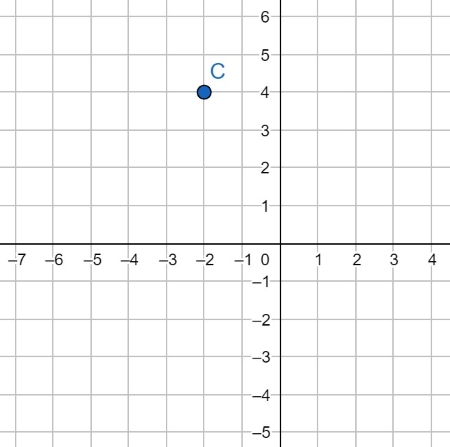Step 2: Create a table with two {eq}x {/eq} values that are less than the {eq}x {/eq} value of the vertex and two {eq}x {/eq} values that are greater than the {eq}x {/eq} value of the vertex. This would give a total of five values of {eq}x {/eq} including the vertex.

xy
-4
-3
-24
-1
0

Step 3: Solve for the corresponding values of {eq}f(x) {/eq} using the chosen values of {eq}x {/eq} to finish the table. Use the first value of {eq}x {/eq}in the table. Substitute this value into the function to determine the output of the {eq}x {/eq} value. Simplify the resulting expression.

\begin{align} f(-4)&=-3(-4+2)^2+4\\ f(-4)&=-3(-2)^2+4\\ f(-4)&=-3(4)+4\\ f(-4)&=-12+4\\ f(-4)&=-8 \end{align}

Repeat this process with the rest of the values of {eq}x {/eq} from the table.

\begin{align} f(-3)&=-3(-3+2)^2+4\\ f(-3)&=-3(-1)^2+4\\ f(-3)&=-3(1)+4\\ f(-3)&=-3+4\\ f(-3)&=1 \end{align}

\begin{align} f(-1)&=-3(-1+2)^2+4\\ f(-1)&=-3(1)^2+4\\ f(-1)&=-3(1)+4\\ f(-1)&=-3+4\\ f(-1)&=1 \end{align}

\begin{align} f(0)&=-3(0+2)^2+4\\ f(0)&=-3(2)^2+4\\ f(0)&=-3(4)+4\\ f(0)&=-12+4\\ f(0)&=-8 \end{align}

We could now fill up the table with the corresponding values of {eq}y {/eq}.

xy
-4-8
-31
-24
-11
0-8

Step 4: Graph the points from the table and connect these points with a smooth curve.

The figures below show the scatterplot and corresponding graph.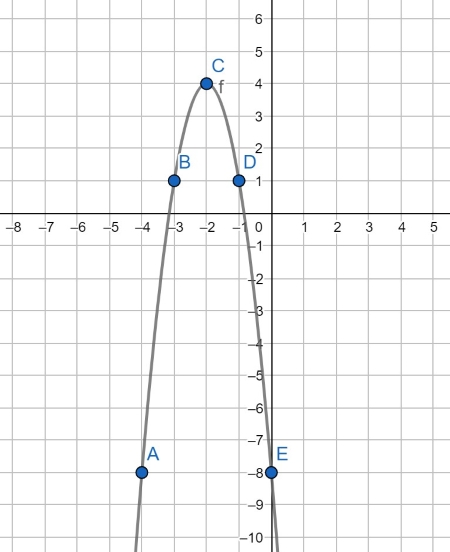## How to Graph a Parabola in Vertex Form: Example 2

Graph the function {eq}f(x)=2(x-5)^2-1 {/eq}

Step 1: Use the vertex form provided to determine the vertex of the function. Graph this point.

For the given function {eq}h=5 {/eq} and {eq}k=-1 {/eq}, that gives us the vertex of the function as {eq}(5,-1) {/eq}. This point is graphed on the coordinate plane below.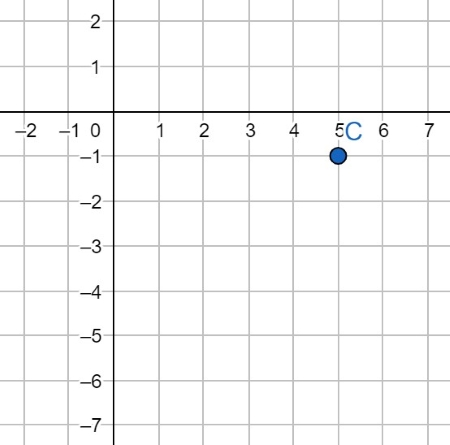Step 2: Create a table with two {eq}x {/eq} values that are less than the {eq}x {/eq} value of the vertex and two {eq}x {/eq} values that are greater than the {eq}x {/eq} value of the vertex. This would give a total of five values of {eq}x {/eq} including the vertex.

xy
3
4
5-1
6
7

Step 3: Solve for the corresponding values of {eq}f(x) {/eq} or {eq}y {/eq} using the values of {eq}x {/eq} in the table.

\begin{align} f(3)&=2(3-5)^2-1\\ f(3)&=2(-2)^2-1\\ f(3)&=2(4)-1\\ f(3)&=8-1\\ f(3)&=7 \end{align}

\begin{align} f(4)&=2(4-5)^2-1\\ f(4)&=2(-1)^2-1\\ f(4)&=2(1)-1\\ f(4)&=2-1\\ f(4)&=1 \end{align}

\begin{align} f(6)&=2(6-5)^2-1\\ f(6)&=2(1)^2-1\\ f(6)&=2(1)-1\\ f(6)&=2-1\\ f(6)&=1 \end{align}

\begin{align} f(7)&=2(7-5)^2-1\\ f(7)&=2(2)^2-1\\ f(7)&=2(4)-1\\ f(7)&=8-1\\ f(7)&=7 \end{align}

Filling up the table, we would have

xy
37
41
5-1
61
77

Step 4: Graph the points from the table and connect these points with a smooth curve.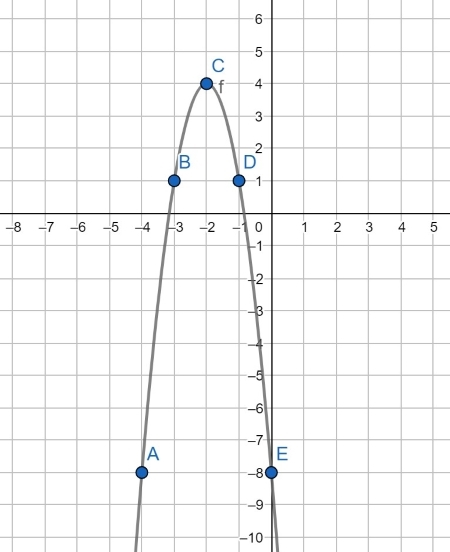Support